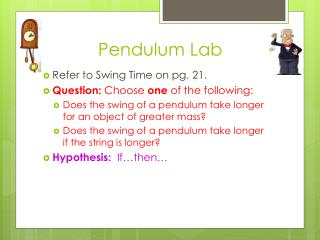DownloadDownload PresentationPendulum Lab

# Pendulum Lab

Download Presentation## Pendulum Lab

- - - - - - - - - - - - - - - - - - - - - - - - - - - E N D - - - - - - - - - - - - - - - - - - - - - - - - - - -
##### Presentation Transcript

1. Pendulum Lab • Refer to Swing Time on pg. 21. • Question: Choose one of the following: • Does the swing of a pendulum take longer for an object of greater mass? • Does the swing of a pendulum take longer if the string is longer? • Hypothesis: If…then…

2. Experiment: • MV: • RV: • CV: (at least 3 important ones) • Materials: • Procedure: (numbered list) – don’t forget multiple trials; quantitative data; start each with a verb • Apparatus setup picture or drawing • Data Table

3. Effect of Length of String on Pendulum Swing Time

4. Effect of Mass on Pendulum Swing Time

5. Analysis: • Average data– round to nearest tenth. • Make a line graph – use graph paper; put the MV on the X-axis (horizontal) and the RV on the Y-axis (vertical). Include labels and scales (use equal intervals) on each axis. Include a title. • Conclusion: • Does the data support the hypothesis? • Answer the question using the data. • Problems. • Extension.